# Python | How to make a terminal progress bar using tqdm

Whether you’re installing software, loading a page or doing a transaction, it always eases your mind whenever you see that small progress bar giving you an estimation of how long the process would take to complete or render. If you have a simple progress bar in your script or code, it looks very pleasing to the eye and gives proper feedback to the user whenever he executes the code. You can use the Python external library tqdm, to create simple & hassle-free progress bars which you can add in your code and make it look lively!

## Installation

Open your command prompt or terminal and type:

`pip install tqdm`

If you are using Python3 then type:

`pip3 install tqdm`

This command would successfully install the library on your computer and is now ready to use.

## Usage

Using `tqdm` is very simple, you just need to add your code between `tqdm()` after importing the library in your code. You need to make sure that the code you put in between the tqdm() function must be iterable or it would not work at all.

Let us see the following example that would help you understand better:

Example:

 `from` `tqdm ``import` `tqdm ` ` `  ` `  `for` `i ``in` `tqdm(``range``(``int``(``9e6``))): ` `    ``pass`

Output: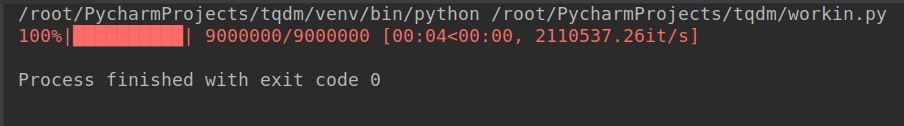Now that we know how to implement tqdm, let’s take a look at some of the important parameters it offers and how it can be used to tweak the progress bar.

• desc: You can use this parameter to specify the description of your progress bar as follows:

Syntax:

`tqdm (self, iterable, desc= “Text You want”)`

Example:

 `from` `tqdm ``import` `tqdm ` `from` `time ``import` `sleep ` ` `  ` `  `for` `i ``in` `tqdm(``range``(``0``, ``100``), desc ``=``"Text You Want"``): ` `    ``sleep(.``1``) `

Output: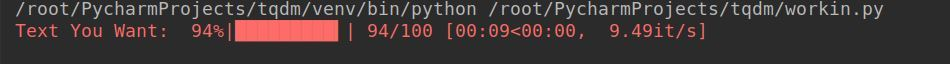• total: This is used to specify the total number of expected iterations if not specified already or needs modification.

Syntax:

`tqdm (self, iterable, total= 500)`

Example:

 `from` `tqdm ``import` `tqdm ` `from` `time ``import` `sleep ` ` `  ` `  `for` `i ``in` `tqdm(``range``(``0``, ``100``), total ``=` `500``, ` `              ``desc ``=``"Text You Want"``): ` `    ``sleep(.``1``) `

Output: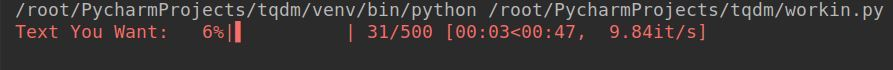• disable: This parameter can be used if you want to completely disable the progress bar.

Syntax:

`tqdm (self, iterable, disable=True)`

Example:

 `from` `tqdm ``import` `tqdm ` `from` `time ``import` `sleep ` ` `  ` `  `for` `i ``in` `tqdm(``range``(``0``, ``100``), disable ``=` `True``, ` `               ``desc ``=``"Text You Want"``): ` `    ``sleep(.``1``) ` ` `  `print``(``"Iteration Successful"``) `

Output: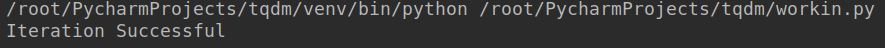• ncols: This parameter is used to specify the entire width of the output message. If left unspecified it remains dynamic to the size of the window. This can be fixed through the `ncols` parameter.

Syntax:

`tqdm (self, iterable, ncols= 100)`

Example:

 `from` `tqdm ``import` `tqdm ` `from` `time ``import` `sleep ` ` `  ` `  `for` `i ``in` `tqdm(``range``(``0``, ``100``), ncols ``=` `100``, ` `               ``desc ``=``"Text You Want"``): ` `    ``sleep(.``1``) `

Output: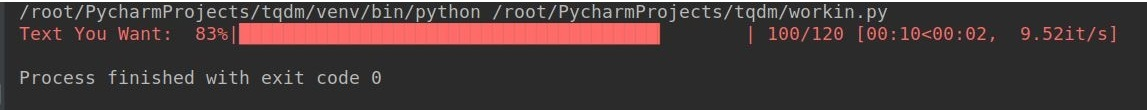• mininterval: You can easily change the minimum progress display update using this option. The default is to 0.1 seconds.

Syntax:

`tqdm (self, iterable, mininterval=3)`

Example:

 `from` `tqdm ``import` `tqdm ` `from` `time ``import` `sleep ` ` `  ` `  `for` `i ``in` `tqdm(``range``(``0``, ``100``), mininterval ``=` `3``,  ` `              ``desc ``=``"Text You Want"``): ` `    ``sleep(.``1``) `

Output: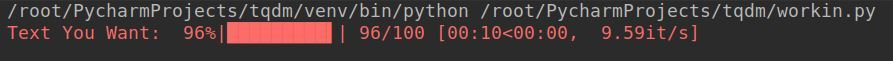• ascii: You can use ASCII characters to fill the progress bar as per your liking.

Syntax:

tqdm (self, iterable, ascii= “123456789\$”, desc=”Text You Want”)

Example:

 `from` `tqdm ``import` `tqdm ` `from` `time ``import` `sleep ` ` `  ` `  `for` `i ``in` `tqdm(``range``(``0``, ``100``),  ` `              ``ascii ``=``"123456789\$"``): ` `    ``sleep(.``1``) `

Output: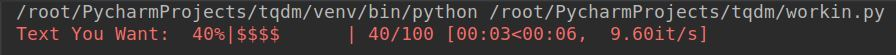• unit: The default unit of time is “it” and can be changed by using this parameter to your preferred unit.

Syntax:

`tqdm (self, iterable, unit= “ ticks”)`

Example:

 `from` `tqdm ``import` `tqdm ` `from` `time ``import` `sleep ` ` `  ` `  `for` `i ``in` `tqdm(``range``(``0``, ``100``), unit ``=``" ticks"``,  ` `              ``desc ``=``"Text You Want"``): ` `    ``sleep(.``1``) `

Output: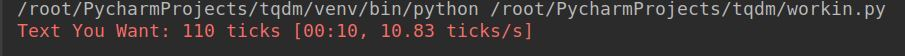• initial
The initial value of the progress bar starts from 0. If you wish to change this, you can use this parameter to initialize the progress bar from the value you wish
Syntax:

`tqdm (self, iterable, initial=50)`

Example:

 `from` `tqdm ``import` `tqdm ` `from` `time ``import` `sleep ` ` `  ` `  `for` `i ``in` `tqdm(``range``(``0``, ``100``), initial ``=` `50``,  ` `              ``desc ``=``"Text You Want"``): ` `    ``sleep(.``1``) `

Output: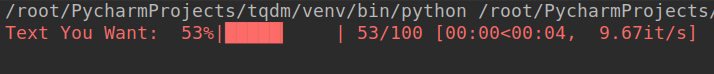The counter would start from 50 and the progress bar would disappear after the final counter is reached. The loop would still run until the iteration is complete.

My Personal Notes arrow_drop_upCheck out this Author's contributed articles.

If you like GeeksforGeeks and would like to contribute, you can also write an article using contribute.geeksforgeeks.org or mail your article to contribute@geeksforgeeks.org. See your article appearing on the GeeksforGeeks main page and help other Geeks.

Please Improve this article if you find anything incorrect by clicking on the "Improve Article" button below.